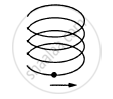Share

# A Particle is Going in a Spiral Path as Shown in Figure with Constant Speed. - Physics

ConceptCircular Motion

#### Question

A particle is going in a spiral path as shown in figure with constant speed.• The velocity of the particle is constant.

• The acceleration of the particle is constant.

• The magnitude of acceleration is constant.

• The magnitude of acceleration is decreasing continuously.

#### Solution

The magnitude of acceleration is constant.

As the pitch and radius of the path is constant, it shows that the magnitude of tangential and radial acceleration is also constant.
Hence, the magnitude of total acceleration is constant.

Is there an error in this question or solution?

#### APPEARS IN

Solution A Particle is Going in a Spiral Path as Shown in Figure with Constant Speed. Concept: Circular Motion.
S